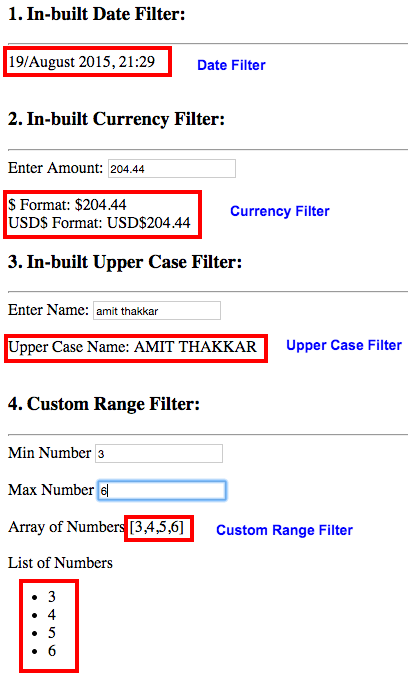# Filters in AngularJS

A filter in AngularJS formats the value of expression to display.

Before moving further let’s remember the line given below:

Whenever you want to show data in a particular format, use filters!

By word, format here we mean a lot of things, some of them listed below:

1. Date Format : Suppose you want to show data in particular format say ‘dd/MMMM yyyy, HH:mm’ in your application and you need to use it at lot of places.

2. Change Format of Text : You want to change the case of text provided by you, say anything provide in lowercase should change to uppercase

3. Range : You need to generate set of numbers within a particular range.

4. Search : Suppose you have following set of data and you want to search to be applied on the below data using Father’s Name only or maybe both Name and Father’s Name:

Name Father’s Name
Nancy Geoffery
Peter John
Kevin Peter

I have listed only handful of use cases, there are plethora of them, where filters can be quite handy.

There are basically 2 types of filters:

1. In-built
2. Custom

Before we see both of these types, let us first see the syntax. Filters are written in view in following manner:

Let’s see a demo given below:

``````Enter Amount: <input type="text" ng-model="data.amount"><br/><br/>
\$ Format: <br/>
USD\$ Format:
``````

In the above demo, we have used an in-built filter named as currency. This filter takes the symbol as its argument. So when we inout some amount in the text box, it shows the amount in a formatted manner with symbol of the currency appended to it.

Now, let us make our own custom filter.

We had discussed a use case, where we might have to generate numbers between a particular range. This can be done using our own custom filter

Here we go:

``````<label>Min Number</label> <input type="text" ng-model="data.min"><br><br>
<label>Max Number</label> <input type="text" ng-model="data.max"><br><br>
<label>Array of Numbers</label> <br><br>
<label>List of Numbers</label>
<ul>
<li ng-repeat="n in data.min | range : data.max"></li>
</ul>
<script type="application/javascript">
var myApp = angular.module('myApp', []);
myApp.filter('range', function () {
return function (min, max) {
if (min > max || max === undefined) {
var temp = max;
max = min;
min = temp || 1;
}
min = parseInt(min);
max = parseInt(max);
var input = [];
for (var i = min; i <= max; i++) {
input.push(i);
}
return input;
}
});
</script>
``````

Let’s what code given above is doing:

1. We have created two input boxes which take min and max number from the user.
2. On the basis of min and max number entered by the user, a list displays all the numbers staring from min number to max number.Now, let’s check how this code works:

1. We take min and max input from user which defines our range.
2. If you see the code given above, then you will notice that we have used a filter named as ‘range’ here.
3. Now, let’s see our JavaScript code. Here we have created a module and then using that module we have defined a filter. Inside the filter function we pass name of the filter as the argument and also define a function which returns another function.
4. It is this returned function that actually does all the work and returns us the formatted result.
5. This function which is returned, takes arguments in the following convention:
 A. 1st argument –> Expression to the left of ‘ ’ where ‘filter’ is declared on the view.

B. 2nd argument –> Expression to the right of the ‘:’ where ‘filter’ is declared on the view.

C. A filter can take ‘n’ number of arguments.

1. For our filter ‘range’, we have taken only 2 arguments - min and max.
2. To min, data.min is passed while max gets data.max.
3. We have parsed the min and max passed to integers and then there is an input array to which all the numbers between min and max is passed.
4. input array is being returned.
5. On view, the entire expression inside is replaced by what is returned by our filter ‘range’, hence we get to see the array containing the numbers.
6. In the rest of the code, we have also created a list of these numbers using a very powerful AngularJS directive ng-repeat.

In this blog we have seen some basic stuff related to filters, there are a hell lot of situations where filters can be used, you just need to identify those situations and use this ultra cool feature of AngularJS!